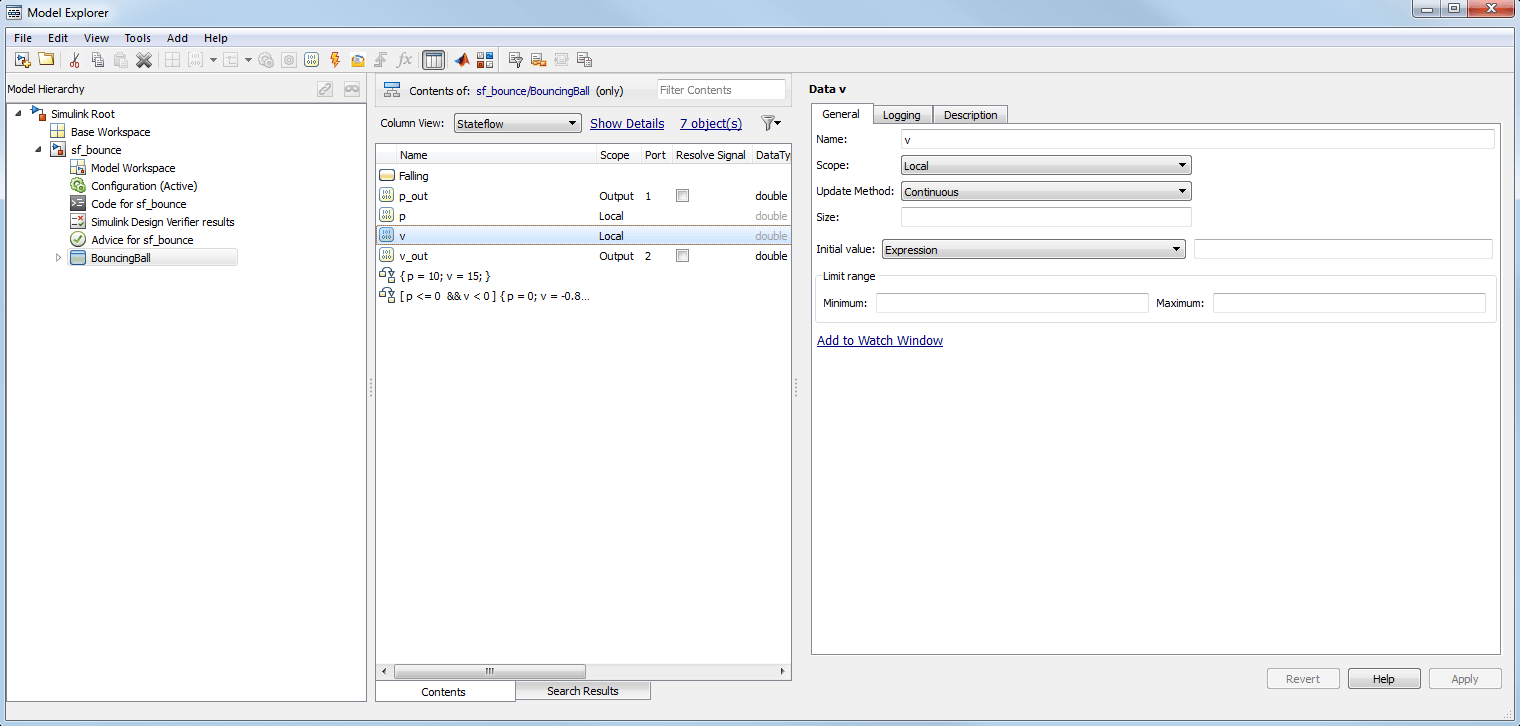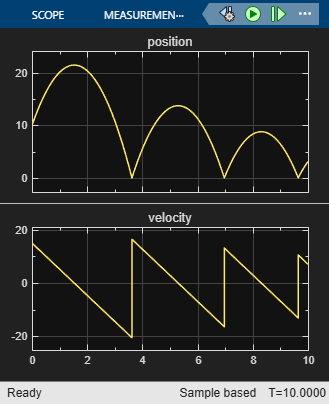# Model a Bouncing Ball in Continuous Time

This example shows how to configure a Stateflow® chart that simulates a bouncing ball in continuous time. The ball moves continuously through the air until it hits the ground, at which point a discontinuity occurs. As a result, the ball suddenly changes direction and velocity. For more information, see Continuous-Time Modeling in Stateflow.

The model sf_bounce contains a chart that updates in continuous-time. Local variables describe the dynamics of a free-falling ball in terms of position and velocity. During simulation, the model uses zero-crossing detection to determine when the ball hits the ground.

### Dynamics of a Bouncing Ball

You can specify how a ball falls freely under the force of gravity in terms of position p and velocity v with this system of first-order differential equations.When p <= 0, the ball hits the ground and bounces. You can model the bounce by updating the position and velocity of the ball:

• Reset the position to p = 0.

• Reset the velocity to the negative of its value just before the ball hit the ground.

• To account for energy loss, multiply the new velocity by a coefficient of distribution (-0.8).

### Configure Chart for Continuous-Time Simulation

In the model, the BouncingBall chart implements modal logic to simulate the continuous dynamics of free fall and the discrete changes associated with bouncing. In the Chart properties dialog box, these settings enable the BouncingBall chart to simulate in continuous time:

• Update method is Continuous so the chart employs continuous-time simulation to model the dynamics of the bouncing ball.

• Enable zero-crossing detection is selected so the Simulink® solver can determine exactly when the ball hits the ground. Otherwise, the Simulink model cannot simulate the physics accurately and the ball appears to descend below ground.### Define Continuous-Time Variables

The BouncingBall chart has two continuous-time variables: p for position and v for velocity. For each one of these variables:

• Scope is Local.

• Type is double.

• Update Method is Continuous.

To expose the continuous state of the chart to the Simulink model, the BouncingBall chart has two output variables: p_out and v_out. For each one of these variables:

• Scope is Output.

• Type is double.

• Update Method is Discrete.

The chart defines the time derivative of continuous-time variables implicitly:

• p_dot is the derivative of position p.

• v_dot as the derivative of velocity v.

In the Model Explorer, you can view the continuous-time local variables and the corresponding outputs in the chart. Implicit derivative variables do not appear in the Model Explorer or in the Symbols pane.### Model Continuous Dynamics of Free Fall

The BouncingBall chart consists of a single state Falling that numerically solves the differential equations for free fall. The default transition into the state sets the initial position to 10 m and the initial velocity to 15 m/s. The during actions in the state:

• Define the derivatives of position and velocity.

• Assign the values of the position and velocity of the ball to the output variables p_out and v_out.### Model Discrete Effects of the Bounce

The Falling state has a self-loop transition that models the discontinuity of the bounce as an instantaneous mode change when the ball suddenly reverses direction. The condition on the transition determines when the ball hits the ground by checking its position p <= 0 and velocity v < 0. If the condition is valid, the condition action resets the position and velocity when the ball hits the ground.

Why Not Check for p == 0?

The ball hits the ground when position p is exactly zero. By relaxing the condition, you increase the tolerance within which the Simulink solver can detect when the position changes sign.

Why Check for v < 0?

The second part of the condition helps maintain the efficiency of the Simulink solver by minimizing the frequency of zero crossings. Without the second check, the condition remains true after the state transition, resulting in two successive zero crossings.

### Validate Chart Semantics

The BouncingBall chart meets the design requirements defined in Guidelines for Continuous-Time Simulation. In particular, the chart:

• Initializes the local variables p and v on the default transition.

• Assigns values to the derivatives p_dot and v_dot in a during action.

• Writes to local variables p and v in a transition action.

• Does not contain events, inner transitions, event-based temporal logic, or change detection operators.

### View Simulation Results

After you run the model, the scope shows the graphs of position and velocity. The position graph exhibits the expected bounce pattern.## SupportGet trial now